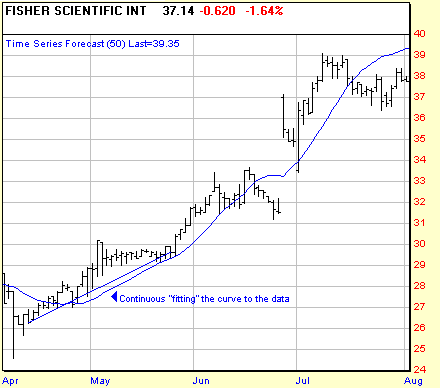# Time Series Forecast

Also known as the moving linear regression indicator or the regression oscillator, the Time Series Forecast indicator illustrates the statistical trend of a security’s price over a given time period. The trend is based on linear regression analysis. The Time Series Forecast plots the last point of multiple linear regression trendlines. The Time Series Forecast does not exhibit as much delay as a Moving Average when adjusting to price changes.Chart courtesy of Prophet Financial Systems (www.prophet.net)